In a previous WIN-Statik Tips & Tricks post, we explained three methods for calculating the buckling of concrete according to EC2. These were the General Method according to EC2 5.8.6, the simplified Stiffness Method 5.8.7 and the Curvature Method 5.8.8.

Questions from Users continue to come though to our Support regarding which of these three methods to use and whether it is possible to combine different methods in terms of instability in different directions.

In this post we provide further consideration to calculating buckling of concrete in order to help explain the appropriate Method.

### Which Method to follow for calculating the buckling of concrete?

Which method to use is, of course, up to you the User. The General Method is considered the most advanced. In this method, a real 2nd order analysis is made simultaneously with a non-linear calculation of how the cracking of the concrete affects the stiffness.

The other two methods are simplified ones, using calibrated formulas of the General method to calculate the 2nd order element. All three methods are allowed according to the Swedish annex.

As mentioned earlier, you can get quite different results depending on the current example. The stiffness method is, for example, very dependent on the amount of reinforcement. With a small reinforcement percentage, one can obtain a significantly lower capacity for this method compared to the others. However, if one increases the reinforcement, the result for the stiffness method will usually be on par with the others.

It is also essential to consider the effective creep, which often has a slightly greater impact on the Stiffness and the General method than the Curvature method.

### Calculating using WIN-Statik Concrete Column and Frame Analysis

When using WIN-Statik, there are two modules that can handle buckling of concrete, Concrete Column, and Frame Analysis. Concrete Column enables calculations according to the two simplified methods, while Frame Analysis can handle all three.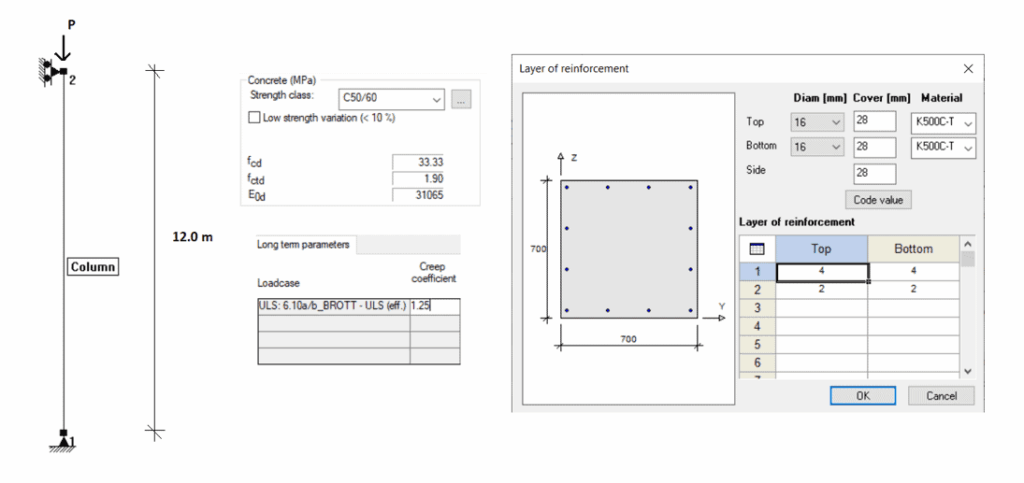Example: To illustrate what this can look like, we have shown and analysed an example of a concrete column 700x700mm with a length of 12m.

The reinforcement content is 12 bars with a diameter of 16 mm, i.e., 2 413 mm2 or 0.492%.

When choosing the stiffness method, we obtain a capacity of 4 865 kN.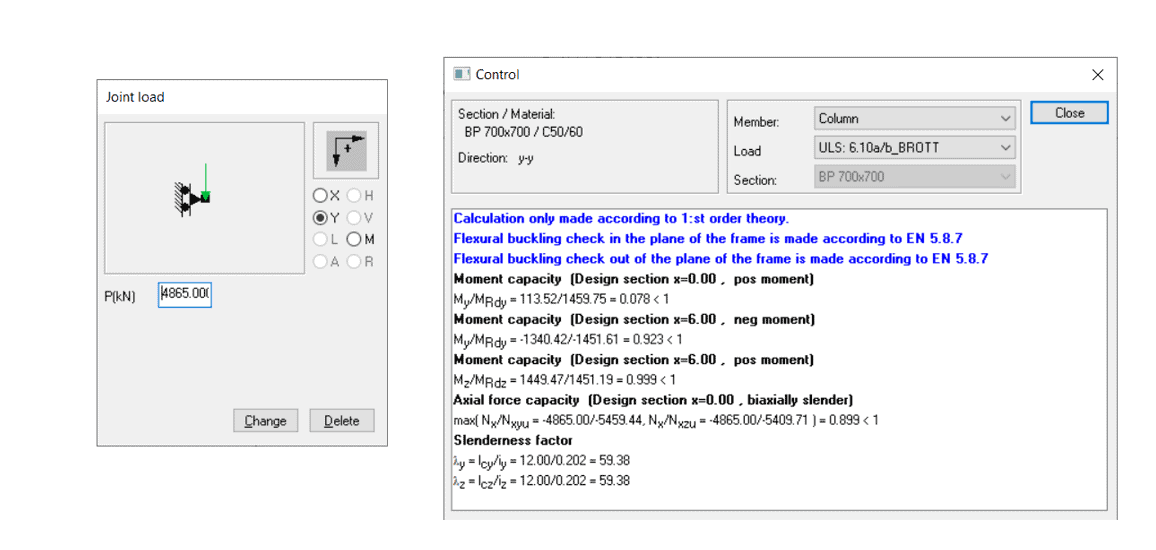Using the curvature method instead, we obtain a capacity of 13 250 kN.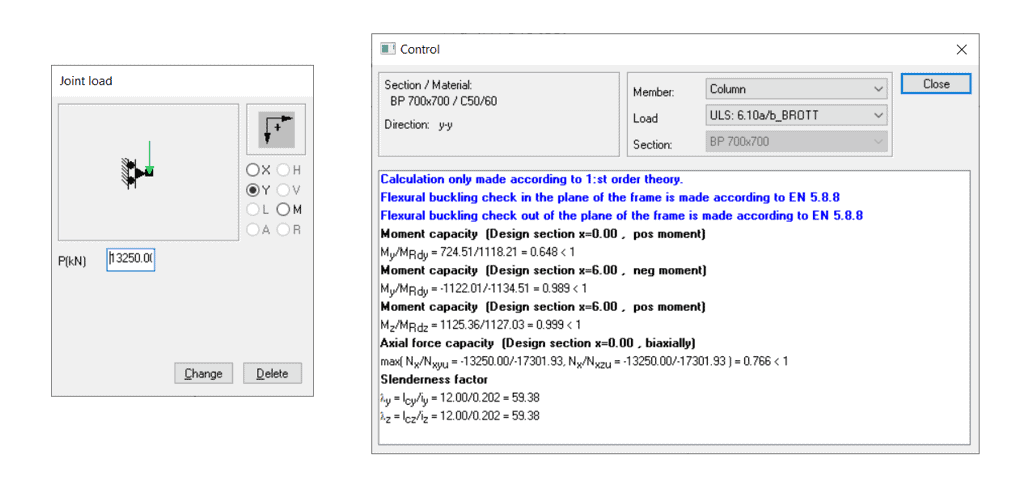Finally, if we use the general method in the plane, the capacity will be 9 265 kN. Out of the plane, one of the simplified methods must be used, in this case, the Stiffness method.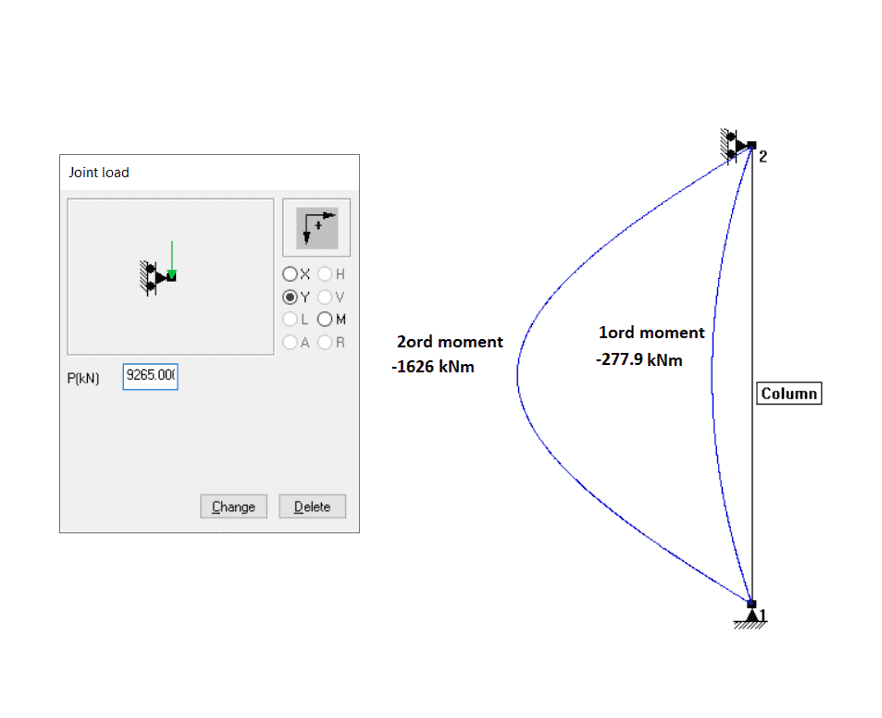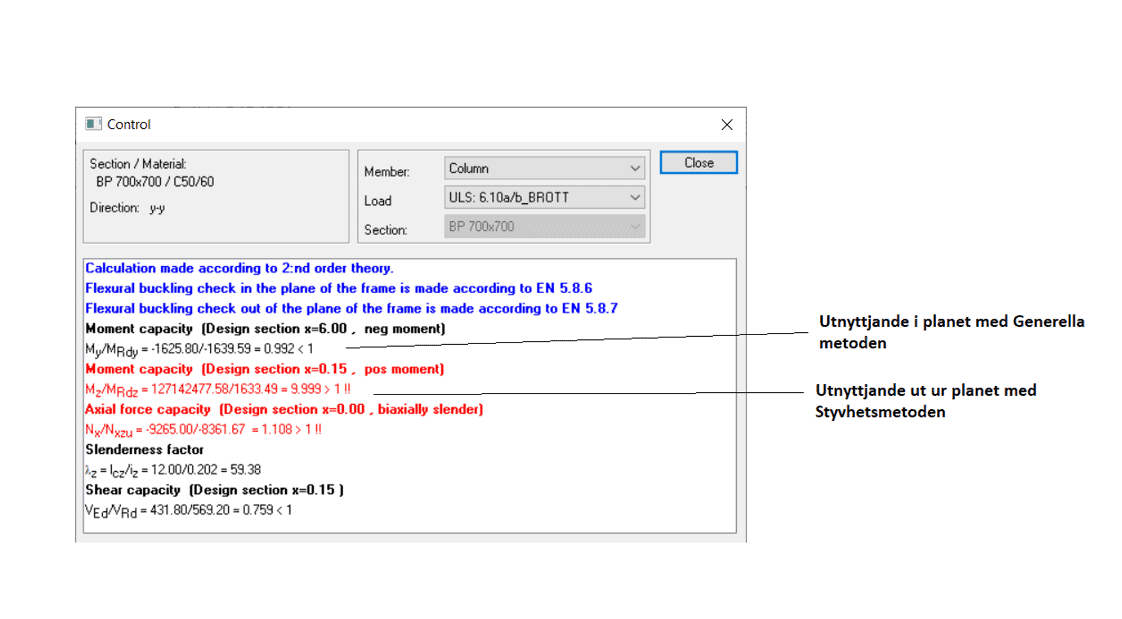If we increase the effective creep to 2.0, the following happens:

The capacity for the Stiffness method decreases to 3 750 kN, i.e., by 23%. For the Curvature method, 12 500 kN, a decrease of 5.3%, and finally, the General method gives 7 835 kN, a decrease of 15.4%.

We go back to the effective crystal number 1.2 and increase the reinforcement significantly to 44 bars with a diameter of 25 mm, i.e., 21 600 mm2 or 4.4%.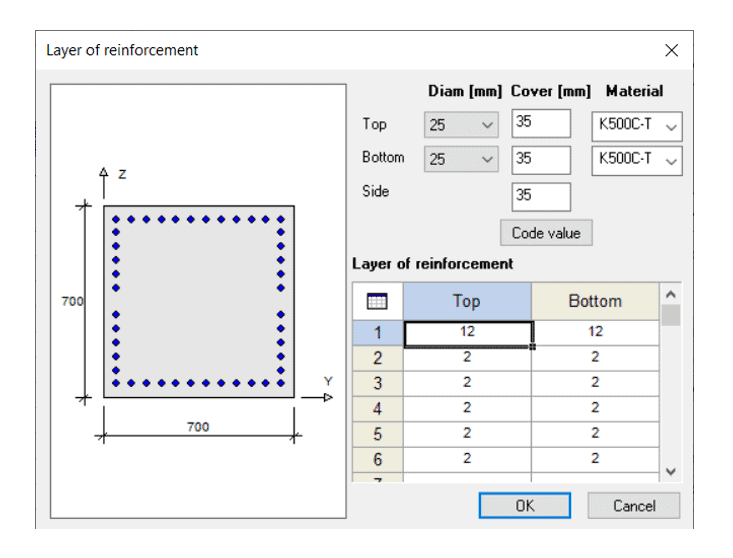The stiffness method now gives 17 880 kN, an increase of 367% from the original value of 4 865 kN. The curvature method gives 19 900 kN, an increase of about 50%, i.e., still slightly more favourable than the Stiffness method, but now the difference is only about 10%. In this case, the General method gives the lowest capacity of 15 415 kN, an increase of about 63%.

### Conclusions

The different methods for calculating bending buckling of concrete can thus strike quite differently, and in this case, it was the curvature method that was most favourable. But, it does not always have to be that way. On the other hand, our experience is that the Stiffness method is usually the most unfavourable at a low reinforcement percentage. Most often, the effective creep affects the Stiffness method and the General method somewhat more.

Our experience is that most calculations are made with the 2nd order analysis, which means the General method in the plane and then the Stiffness or Curvature method according to the User’s choice.

### WIN-Statik Wiki

Full technical details and the theory behind the software can be found on the WIN-Statik Wiki by clicking here.